Updating search results...

# 590 Results

View
Selected filters:
• Open Middle MathRestricted Use
Rating
0.0 stars

Open Middle provides math problems that have a closed beginning, a closed end, and an open middle. This means that there are multiple ways to approach and ultimately solve the problems. Open middle problems generally require a higher Depth of Knowledge than most problems that assess procedural and conceptual understanding.

Subject:
Mathematics
Material Type:
Activity/Lab
Provider:
Open Middle Math
04/26/2022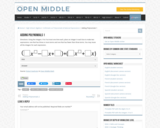Restricted Use
Rating
0.0 stars

Open Middle provides math problems that have a closed beginning, a closed end, and an open middle. This means that there are multiple ways to approach and ultimately solve the problems. Open middle problems generally require a higher Depth of Knowledge than most problems that assess procedural and conceptual understanding.

Subject:
Mathematics
Material Type:
Activity/Lab
Provider:
Open Middle Math
04/26/2022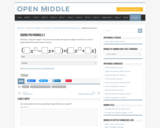Restricted Use
Rating
0.0 stars

Open Middle provides math problems that have a closed beginning, a closed end, and an open middle. This means that there are multiple ways to approach and ultimately solve the problems. Open middle problems generally require a higher Depth of Knowledge than most problems that assess procedural and conceptual understanding.

Subject:
Mathematics
Material Type:
Activity/Lab
Provider:
Open Middle Math
04/26/2022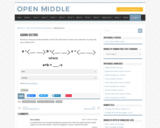Restricted Use
Rating
0.0 stars

Open Middle provides math problems that have a closed beginning, a closed end, and an open middle. This means that there are multiple ways to approach and ultimately solve the problems. Open middle problems generally require a higher Depth of Knowledge than most problems that assess procedural and conceptual understanding.

Subject:
Mathematics
Material Type:
Activity/Lab
Provider:
Open Middle Math
04/26/2022Restricted Use
Rating
0.0 stars

Open Middle provides math problems that have a closed beginning, a closed end, and an open middle. This means that there are multiple ways to approach and ultimately solve the problems. Open middle problems generally require a higher Depth of Knowledge than most problems that assess procedural and conceptual understanding.

Subject:
Mathematics
Material Type:
Activity/Lab
Provider:
Open Middle Math
04/26/2022Conditional Remix & Share Permitted
CC BY-SA
Rating
0.0 stars

Open Middle provides math problems that have a closed beginning, a closed end, and an open middle. This means that there are multiple ways to approach and ultimately solve the problems. Open middle problems generally require a higher Depth of Knowledge than most problems that assess procedural and conceptual understanding.

Subject:
Mathematics
Material Type:
Activity/Lab
Provider:
Open Middle Math
04/26/2022Restricted Use
Rating
0.0 stars

Open Middle provides math problems that have a closed beginning, a closed end, and an open middle. This means that there are multiple ways to approach and ultimately solve the problems. Open middle problems generally require a higher Depth of Knowledge than most problems that assess procedural and conceptual understanding.

Subject:
Mathematics
Material Type:
Activity/Lab
Provider:
Open Middle Math
04/26/2022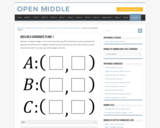Restricted Use
Rating
0.0 stars

Open Middle provides math problems that have a closed beginning, a closed end, and an open middle. This means that there are multiple ways to approach and ultimately solve the problems. Open middle problems generally require a higher Depth of Knowledge than most problems that assess procedural and conceptual understanding.

Subject:
Mathematics
Material Type:
Activity/Lab
Provider:
Open Middle Math
04/26/2022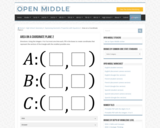Restricted Use
Rating
0.0 stars

Open Middle provides math problems that have a closed beginning, a closed end, and an open middle. This means that there are multiple ways to approach and ultimately solve the problems. Open middle problems generally require a higher Depth of Knowledge than most problems that assess procedural and conceptual understanding.

Subject:
Mathematics
Material Type:
Activity/Lab
Provider:
Open Middle Math
04/26/2022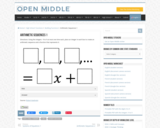Restricted Use
Rating
0.0 stars

Open Middle provides math problems that have a closed beginning, a closed end, and an open middle. This means that there are multiple ways to approach and ultimately solve the problems. Open middle problems generally require a higher Depth of Knowledge than most problems that assess procedural and conceptual understanding.

Subject:
Mathematics
Material Type:
Activity/Lab
Provider:
Open Middle Math
04/26/2022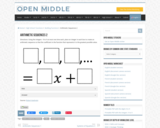Restricted Use
Rating
0.0 stars

Open Middle provides math problems that have a closed beginning, a closed end, and an open middle. This means that there are multiple ways to approach and ultimately solve the problems. Open middle problems generally require a higher Depth of Knowledge than most problems that assess procedural and conceptual understanding.

Subject:
Mathematics
Material Type:
Activity/Lab
Provider:
Open Middle Math
04/26/2022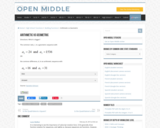Restricted Use
Rating
0.0 stars

Open Middle provides math problems that have a closed beginning, a closed end, and an open middle. This means that there are multiple ways to approach and ultimately solve the problems. Open middle problems generally require a higher Depth of Knowledge than most problems that assess procedural and conceptual understanding.

Subject:
Mathematics
Material Type:
Activity/Lab
Provider:
Open Middle Math
04/26/2022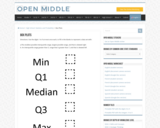Restricted Use
Rating
0.0 stars

Open Middle provides math problems that have a closed beginning, a closed end, and an open middle. This means that there are multiple ways to approach and ultimately solve the problems. Open middle problems generally require a higher Depth of Knowledge than most problems that assess procedural and conceptual understanding.

Subject:
Mathematics
Material Type:
Activity/Lab
Provider:
Open Middle Math
04/26/2022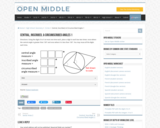Restricted Use
Rating
0.0 stars

Open Middle provides math problems that have a closed beginning, a closed end, and an open middle. This means that there are multiple ways to approach and ultimately solve the problems. Open middle problems generally require a higher Depth of Knowledge than most problems that assess procedural and conceptual understanding.

Subject:
Mathematics
Material Type:
Activity/Lab
Provider:
Open Middle Math
04/26/2022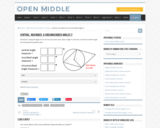Restricted Use
Rating
0.0 stars

Open Middle provides math problems that have a closed beginning, a closed end, and an open middle. This means that there are multiple ways to approach and ultimately solve the problems. Open middle problems generally require a higher Depth of Knowledge than most problems that assess procedural and conceptual understanding.

Subject:
Mathematics
Material Type:
Activity/Lab
Provider:
Open Middle Math
04/26/2022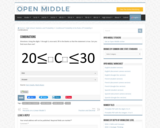Restricted Use
Rating
0.0 stars

Open Middle provides math problems that have a closed beginning, a closed end, and an open middle. This means that there are multiple ways to approach and ultimately solve the problems. Open middle problems generally require a higher Depth of Knowledge than most problems that assess procedural and conceptual understanding.

Subject:
Mathematics
Material Type:
Activity/Lab
Provider:
Open Middle Math
04/26/2022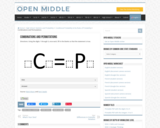Restricted Use
Rating
0.0 stars

Open Middle provides math problems that have a closed beginning, a closed end, and an open middle. This means that there are multiple ways to approach and ultimately solve the problems. Open middle problems generally require a higher Depth of Knowledge than most problems that assess procedural and conceptual understanding.

Subject:
Mathematics
Material Type:
Activity/Lab
Provider:
Open Middle Math
04/26/2022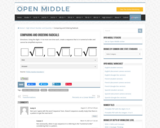Restricted Use
Rating
0.0 stars

Open Middle provides math problems that have a closed beginning, a closed end, and an open middle. This means that there are multiple ways to approach and ultimately solve the problems. Open middle problems generally require a higher Depth of Knowledge than most problems that assess procedural and conceptual understanding.

Subject:
Mathematics
Material Type:
Activity/Lab
Provider:
Open Middle Math
04/26/2022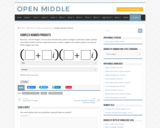Restricted Use
Rating
0.0 stars

Open Middle provides math problems that have a closed beginning, a closed end, and an open middle. This means that there are multiple ways to approach and ultimately solve the problems. Open middle problems generally require a higher Depth of Knowledge than most problems that assess procedural and conceptual understanding.

Subject:
Mathematics
Material Type:
Activity/Lab
Provider:
Open Middle Math
04/26/2022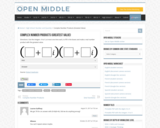Restricted Use